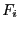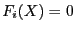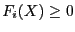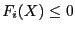Next: Interval Function Up: Implementation Previous: Number of unknowns and   Contents

Type of the functions

The i-th value in the array ofintegers Type_Eq enable one to indicate if functionis an equation or an inequality:
• Type_Eq(i)=0 :must verify• Type_Eq(i)=1 :must verify• Type_Eq(i)=-1 :must verifyFor all the general solving algorithms the integer ALIAS_Pure_Equation is set to the number of equations in the constraints list.

Jean-Pierre Merlet 2012-12-20